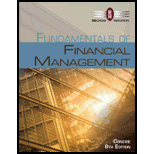# WACC The Patrick Company’s year-end balance sheet is shown below. Its cost of common equity is 16%, its before-tax cost of debt is 13%, and its marginal tax rate is 40%. Assume that the firm’s long-term debt sells at par value. The firm’s total debt, which is the sum of the company’s short-term debt and long-term debt, equals $1,152. The firm has 576 shares of common stock outstanding that sell for$4.00 per share. Calculate Patrick’s WACC using market-value weights. Assets Liabilities and Equity Cash $130 Accounts payable and accruals$ 10 Accounts receivable 240 Short term debt 52 Inventories 360 Long term debt 1,100 Plant and equipment, net 2,160 Common equity 1,1728 Total assets $2,890 Total liabilities and equity$2,890### Fundamentals of Financial Manageme...

8th Edition
Eugene F. Brigham + 1 other
Publisher: Cengage Learning
ISBN: 9781285065137

#### Solutions

Chapter
Section### Fundamentals of Financial Manageme...

8th Edition
Eugene F. Brigham + 1 other
Publisher: Cengage Learning
ISBN: 9781285065137
Chapter 10, Problem 9P
Textbook Problem
25 views

## WACC The Patrick Company’s year-end balance sheet is shown below. Its cost of common equity is 16%, its before-tax cost of debt is 13%, and its marginal tax rate is 40%. Assume that the firm’s long-term debt sells at par value. The firm’s total debt, which is the sum of the company’s short-term debt and long-term debt, equals $1,152. The firm has 576 shares of common stock outstanding that sell for$4.00 per share. Calculate Patrick’s WACC using market-value weights. Assets Liabilities and Equity Cash $130 Accounts payable and accruals$ 10 Accounts receivable 240 Short term debt 52 Inventories 360 Long term debt 1,100 Plant and equipment, net 2,160 Common equity 1,1728 Total assets $2,890 Total liabilities and equity$2,890

Summary Introduction

To calculate: P Company WACC using market value weights.

Weighted Average Cost of Capital:

It is the weighted average cost of capital of all the sources through which firm finances its capital. It is that rate that company will pay to all for raising finance. It can be termed as firm’s cost of capital.

The company raises money through various sources such as common stock, preference share debt the WACC is calculated taking the relative weight of each item of capital structure.

The formula of WACC is:

WACC=Wdrd(1t)+WPrp+Wcrs

Where

• Wd is the weight of the debt.
• WP is the weight of the preferred stock.
• Wc is the weight of the equity.
• rd is cost of the debt.
• rP is cost of the preferred stock.
• rc is the cost of the equity.

### Explanation of Solution

Given,

Before-tax cost of debt is 13%

Weight of debt is 0.3333

Cost of equity is 16%

Weight of equity is 0.6667

The company does not have any preferred stock the WACC can be calculated as

WACC=Wdrd(1t)+Wcrs

Where

• Wd is the weight of the debt.
• Wc is the weight of the equity.
• rd is cost of the debt.
• rc is the cost of the equity.

Substitute 0.3333 for Wd, 0.13 for rd 0.16 for rc and 0.6667 for Wc in above formula.

=0.3333×0.13(10.40)+0.6667×0.16=0.3333×0

### Still sussing out bartleby?

Check out a sample textbook solution.

See a sample solution

#### The Solution to Your Study Problems

Bartleby provides explanations to thousands of textbook problems written by our experts, many with advanced degrees!

Get Started